How to Learn in 24 Hours?The Rapid Learning Movie

 Need Help? M-F: 9am-5pm(PST): Toll-Free: (877) RAPID-10 US Direct: (714) 692-2900 Int'l: 001-714-692-2900 24/7 Online Technical Support: The Rapid Support Center Secure Online Order:Need Proof? Testimonials by Our Users

 Rapid Learning Courses: MCAT in 24 Hours (2015-16) USMLE in 24 Hours (Boards) Chemistry in 24 Hours Biology in 24 Hours Physics in 24 Hours Mathematics in 24 Hours Psychology in 24 Hours SAT in 24 Hours ACT in 24 Hours AP in 24 Hours CLEP in 24 Hours DAT in 24 Hours (Dental) OAT in 24 Hours (Optometry) PCAT in 24 Hours (Pharmacy) Nursing Entrance Exams Certification in 24 Hours eBook - Survival Kits Audiobooks (MP3)

 Tell-A-Friend: Have friends taking science and math courses too? Tell them about our rapid learning system.Home »  Mathematics »  Introductory Statistics

Linear Regression

 Topic Review on "Title": Model: an equation used to characterize the relationship between variables and predict future outcomes. Models: we can specifically model the relationship of variables with a line and its equation. Models use parameters are numbers, or numbers that are derived from the data. Recall that the Normal model was specified with mean µ and standard deviation σ. A linear model is the equation of a straight line through the data.  Similar to the slope-intercept form of a line, y = mx + b. Predicted Value: is the estimate made from a model, known as(y-hat). Residual: the difference between the observed value and the predicted value of an observation, otherwise known as error. Residual =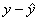Line of Best Fit: The line for which the sum of the squared residuals is minimized, known as the least squares regression line. Residual Plot:  plots residuals on the vertical axis and the explanatory variable on the horizontal axis. Influential Observations: Are those observations that markedly change the position of the regression line. R2, coefficient of determination:  the proportion of variability of y accounted for by the least squares regression on x. Extrapolation: the use of a regression line for prediction outside the domain of values of the explanatory variable we used to obtain the line. Least Squares Regression Equation,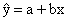Slope,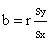Y-intercept,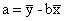Least Squares Regression Line: Also called the best line of fit. This line minimizes residuals between the line and the observed values. The y-intercept is the value of y when x = 0 The slope says that a change of one standard deviation of x corresponds to a change of r standard deviations in y along the regression line In other words, the y units per every x unitRapid Study Kit for "Title":
 Flash Movie Flash Game Flash Card Core Concept Tutorial Problem Solving Drill Review Cheat Sheet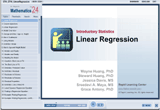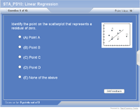"Title" Tutorial Summary : This tutorial shows the definitions of linear regression. Basically, a model is an equation used to characterize the relationship between variables and predict future outcomes. A linear model is the equation of a straight line through the data, similar to the slope-intercept form of a line, y = mx + b. Therefore by completing this tutorial, you will be able to find a linear regression model that best fit the data (minimized the residuals) and thus able to predict future response values or outcomes.

 Tutorial Features: Specific Tutorial Features: Residuals can be used to work out and illustrate the linear regression example problems, step by step. Examples showing how to build a model and find the line of fit are provided. Series Features: Concept map showing inter-connections of new concepts in this tutorial and those previously introduced. Definition slides introduce terms as they are needed. Visual representation of concepts Animated examples—worked out step by step A concise summary is given at the conclusion of the tutorial.

 "Title" Topic List: Model Predicted Value Residual Residual Plot Influential Observations Verify the fit of a regression line Identify, calculate and interpret r2, the coefficient of determination

See all 24 lessons in Introductory Statistics, including concept tutorials, problem drills and cheat sheets:
Teach Yourself Introductory Statistics Visually in 24 Hours